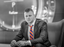top of pageSearch
•William B

# vSAN Memory Consumption Calculations

Updated: Oct 26, 2020I was trying to calculate the vSAN overhead / memory for a 4 node VxRAIL E560F cluster using the manual method. I know that there are several fancy calculators out there, the best one being the vSAN ReadyNode Sizer https://vsansizer.vmware.com/, but I wanted to know the actual math behind it and how the estimated numbers were determined.

In my example, I have a 4 node VxRAIL management cluster, each node has the following configuration:

CPU

VXR INTEL CPU GD 6238 2.1G 22C

1 x Physical CPU = 22 Cores

RAM

VXRAIL MEMORY 32GB 2933MT RDIMM

x12 32 GB RDIMM Modules

Cache Devices for vSAN

VXR MIXED USE 800GB 2.5 CACHE

1 x 800 GB SSD

Capacity Devices for vSAN

VXR 1.92TB CAPACITY SATA 2.5IN SSD

2 x 1.92 TB

There is a KB article out there that gives some details on how to determine memory overhead: https://kb.vmware.com/s/article/2113954. In this article they give an example for how you can calculate an all flash configuration with 1 disc group with the following formula:

HOST_FOOTPRINT + NumDiskGroups * (DISKGROUP_FIXED_FOOTPRINT + DISKGROUP_SCALABLE_FOOTPRINT + CacheSize * CACHE_DISK_FOOTPRINT + NumCapacityDisks * CAPACITY_DISK_FOOTPRINT).

In the beginning of the KB, they give a few constants, which are fixed numbers that are used to make the rest of the equation:

HOST_FOOTPRINT = 7100 MB

CAPACITY_DISK_FOOTPRINT = 160 MB (ALL_FLASH)

CACHE_DISK_FOOTPRINT = 20 MB (ALL_FLASH)

DISKGROUP_FIXED_FOOTPRINT = 1360 MB (ALL_FLASH) *

CAPACITY_DISK_FOOTPRINT = 180 MB (HYBRID)

CACHE_DISK_FOOTPRINT = 10 MB (HYBRID)

DISKGROUP_FIXED_FOOTPRINT = 1610 MB (HYBRID)

DISKGROUP_SCALABLE_FOOTPRINT = 0.5% of system memory **

In the examples they give a host with 256GB memory as an example. Based on the constants the DISKGROUP_SCALABLE_FOOTPRINT is 0.5% of 256GB, which is 1,28GB or 128000MB.But for some reason they have 1310 for their DISKGROUP_SCALABLE_FOOTPRINT.

This confused me and I was not sure how they are getting this number at all, as clearly 0.5% of 256GB is 1,28GB. I was thinking that I had maybe missed something fundamental here, as math had never been my strong point. Upon further investigation I found out what the issue is:

When determining DISKGROUP_SCALABLE_FOOTPRINT, you need to take the example 256GB number and multiply this by 1024, as 1024 is in base 2. 256 * 1024 = 262144.

Then you take 262144 and multiply that by 0.005 to get 1310, which becomes your scalable footprint. The rest of the equation is pretty explanatory.

So going back to my sizing example, here are the vSAN memory calculation for each ESXi host in the cluster:

DISKGROUP_FIXED_FOOTPRINT = 1360MB

DISKGROUP_SCALABLE_FOOTPRINT = 384 * 1024 = 393216 * 0.005 = 1966.08

CacheSize = 800GB

CACHE_DISK_FOOTPRINT = 20 MB

NumCapacityDisks = 2

CAPACITY_DISK_FOOTPRINT = 160 MB

1360 + 1966.08 + 800 * 20 + 2 * 160 = 19646,08

7100 + 1 * 19646,08 = 26.74GB vSAN Footprint or 106,96GB for a 4 node cluster

Also to note, the KB article lists that you can also "enable" Dedup and Compression into your equations:

• For diskgroups that has the compression-only feature turned on, there is an additional cost of 39.5 MB per capacity disk.

• For diskgroups that has the deduplication feature turned on, there is an additional cost of 120 MB per disk group.

These baseline numbers can be used as inputs for other sizing and scaling equations. As I figure those out, I will be sure to update my blog here with the details.

Otherwise just use the sizing tool, it´s a lot easier!

13 views
bottom of page